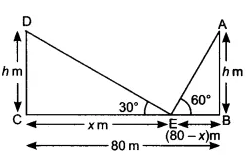Q

# Two poles of equal heights are standing opposite each other on either side of the road, which is 80 m wide. From a point between them on the road, the angles of elevation of the top of the poles are 60° and 30°, respectively. Find the height

10. Two poles of equal heights are standing opposite each other on either side of the road, which is 80 m wide. From a point between them on the road, the angles of elevation of the top of the poles are 60° and 30°, respectively. Find the height of the poles and the distances of the point from the poles.

ViewsGiven that,
The height of both poles are equal  DC = AB. The angle of elevation of of the top of the poles are  and  resp.
Let the height of the poles be   m and CE =  and BE = 80 -

According to question,
In triangle DEC,

..............(i)

In triangle AEB,
..................(ii)
On equating eq (i) and eq (ii), we get

m
So,  = 60 m

Hence the height of both poles is ()m and the position of the point is at 60 m from the pole CD and 20 m from the pole AB.

Exams
Articles
Questions